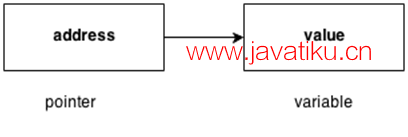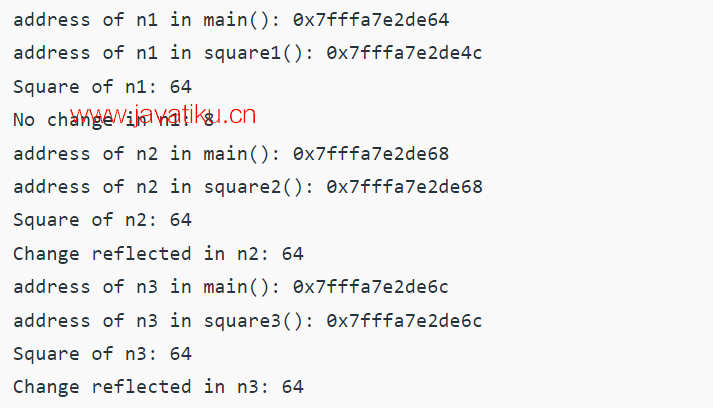# C++教程-C++指针## C++ 指针

C++ 语言中的指针是一种变量，它也被称为定位器或指示器，用于指向一个值的地址。

#### 语法

``````数据类型 *变量名;
int *ptr; // ptr 可以指向一个保存 int 数据的地址 ``````

### 如何使用指针？

1. 声明指针变量。
2. 使用一元运算符 (&) 可以获取变量的地址，将指针赋值为变量的地址。
3. 使用一元运算符 (*) 可以访问提供的地址中变量的值。#### 指针的优点

1. 指针减少了代码并提高了性能，它用于检索字符串、树等，并与数组、结构和函数一起使用。
2. 我们可以使用指针从函数中返回多个值。
3. 它使您能够访问计算机内存中的任何内存位置。

#### 指针的用途

C++ 语言中有许多使用指针的用途。

1. 动态内存分配

1. 数组、函数和结构

C 语言中的指针广泛用于数组、函数和结构。它减少了代码并提高了性能。

### 指针中使用的符号

&（取地址运算符）地址运算符确定变量的地址
*（间接运算符）间接运算符访问给定参数所提供地址的变量的值

### 声明指针

C++ 语言中的指针可以使用 *（星号符号）声明。

``````int *a; // 指向 int 的指针
char *c; // 指向 char 的指针 ``````

### 指针示例

``````#include <iostream>
using namespace std;

int main()
{
int number = 30;
int *p;
p = &number; // 存储 number 变量的地址
cout << "Address of number variable is: " << &number << endl;
cout << "Address of p variable is: " << p << endl;
cout << "Value of p variable is: " << *p << endl;

return 0;
}``````

``Address of number variable is: 0x7ffccc8724c4 Address of p variable is: 0x7ffccc8724c4 Value of p variable is: 30``

### 使用指针交换两个数（不使用第三个变量）

``````#include <iostream>
using namespace std;

int main()
{
int a = 20, b = 10, *p1 = &a, *p2 = &b;
cout << "Before swap: *p1=" << *p1 << " *p2=" << *p2 << endl;
*p1 = *p1 + *p2;
*p2 = *p1 - *p2;
*p1 = *p1 - *p2;
cout << "After swap: *p1=" << *p1 << " *p2=" << *p2 << endl;

return 0;
}``````

``````Before swap: *p1=20 *p2=10
After swap: *p1=10 *p2=20``````

### 什么是无效指针？

``````int *ptr1;
int arr;
int *ptr2 = arr+20;``````

### 什么是空指针（null pointer）？

``````int *ptr1 = 0;
int *ptr2 = NULL;``````

### 什么是指向指针的指针？

``````char a;
char *b;
char **c;
a = 'g';
b = &a;
c = &b;``````

### 引用和指针有什么区别？

1. 值传递
2. 通过指针参数进行引用传递
3. 通过引用参数进行引用传递

#### 示例：

``````#include <iostream>
using namespace std;

// 值传递
int square1(int n)
{
cout << "n1的地址（在square1()内）: " << &n << "\n";
n *= n;
return n;
}

// 指针传递
void square2(int* n)
{
cout << "n2的地址（在square2()内）: " << n << "\n";
*n *= *n;
}

// 引用传递
void square3(int& n)
{
cout << "n3的地址（在square3()内）: " << &n << "\n";
n *= n;
}

void example()
{
// 值传递
int n1 = 8;
cout << "n1的地址（在main()内）: " << &n1 << "\n";
cout << "n1的平方: " << square1(n1) << "\n";
cout << "n1的值未改变: " << n1 << "\n";

// 指针传递
int n2 = 8;
cout << "n2的地址（在main()内）: " << &n2 << "\n";
square2(&n2);
cout << "n2的平方: " << n2 << "\n";
cout << "n2的值发生了改变: " << n2 << "\n";

// 引用传递
int n3 = 8;
cout << "n3的地址（在main()内）: " << &n3 << "\n";
square3(n3);
cout << "n3的平方: " << n3 << "\n";
cout << "n3的值发生了改变: " << n3 << "\n";
}

int main()
{
example();
return 0;
}``````